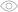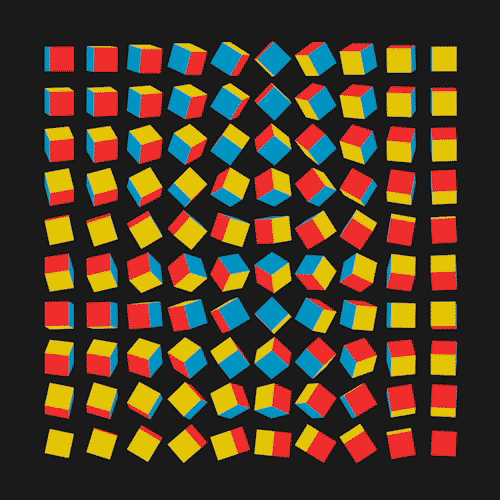# IQ Test: Odd One Out in Figures5056Many adults prefer spatial reasoning in their work - it's necessary for creative and engineering occupations. Regardless of career, a person's ability to operate with visuospatial images helps to solve practical problems. Take the quiz to find out how good you are at geometry and logical problems.

What figure is odd in the row?

All the other figures can be turned upside down without any changes.

1

2

3

4

5

What figure is odd in the row?

All the other figures can be combined by rotating.

1

2

3

4

5

What figure is odd in the row?

All the other figures can be combined by rotating.

1

2

3

4

5

What figure is odd in the row?

All the other figures can be combined by rotating.

1

2

3

4

5

What figure is odd in the row?

All the other figures can be combined by rotating.

1

2

3

4

5

What figure is odd in the row?

All the other figures can be combined by rotating in the place of the picture.

1

2

3

4

What figure is odd in the row?

The different positions of the right hand are depicted in all the other picturesl the third figure depicts the left hand.

1

2

3

4

What figure is odd in the row?

The figure turns every time by a quarter of the semicircle counter-clockwise, in doing so the figure's color changes to the opposite. The exception is figure 3.

1

2

3

4

5

What figure is odd in the row?

A set of four circles every time turns 90 degrees but a circle with a "+" sign is always located in one horizontal row with a black circle regardless of it. The exception is figure 5.

1

2

3

4

5

What figure is odd in the row?

Figures 1 and 3, as well as 2 and 4 are paired, they can be combined with each other rotating in the picture's planes by 90 degrees. Figure 5 cannot be combined with any.

1

2

3

4

5

What figure is odd in the row?

The picture turns 90 degrees every time. The dashed lines in figure 3 are directed in the wrong way.

1

2

3

4

5

Architect with a God-given talent

Aren't you an architect by training? Or maybe a geometry teacher? We'll say one thing doe sure, you did great with our problems and this means your spatial reasoning and logic is well-developed. Keep it up. Share this quiz on social media - let's see how your friends will do.

Geometry fan

You've made a few mistakes but nonetheless your spatial thinking is at quite a high level. At school, you probably got along with geometry, that's why you did your problems to a T. Share this quiz on social media - let's see how your friends will do.

I'm an artist - I see this way

Let's face it, you're pretty bad at spatial thinking. But don't feel down! Even if you cannot be an architect, you can succeed in other areas. For example, sales or IT. Share this quiz on social media - let's see how your friends will do.

What figure is odd in the row?
1 / 11What figure is odd in the row?
2 / 11What figure is odd in the row?
3 / 11What figure is odd in the row?
4 / 11What figure is odd in the row?
5 / 11What figure is odd in the row?
6 / 11What figure is odd in the row?
7 / 11What figure is odd in the row?
8 / 11What figure is odd in the row?
9 / 11What figure is odd in the row?
10 / 11What figure is odd in the row?
11 / 11### Calculating results...

Useful and short ads help us create new content every day.